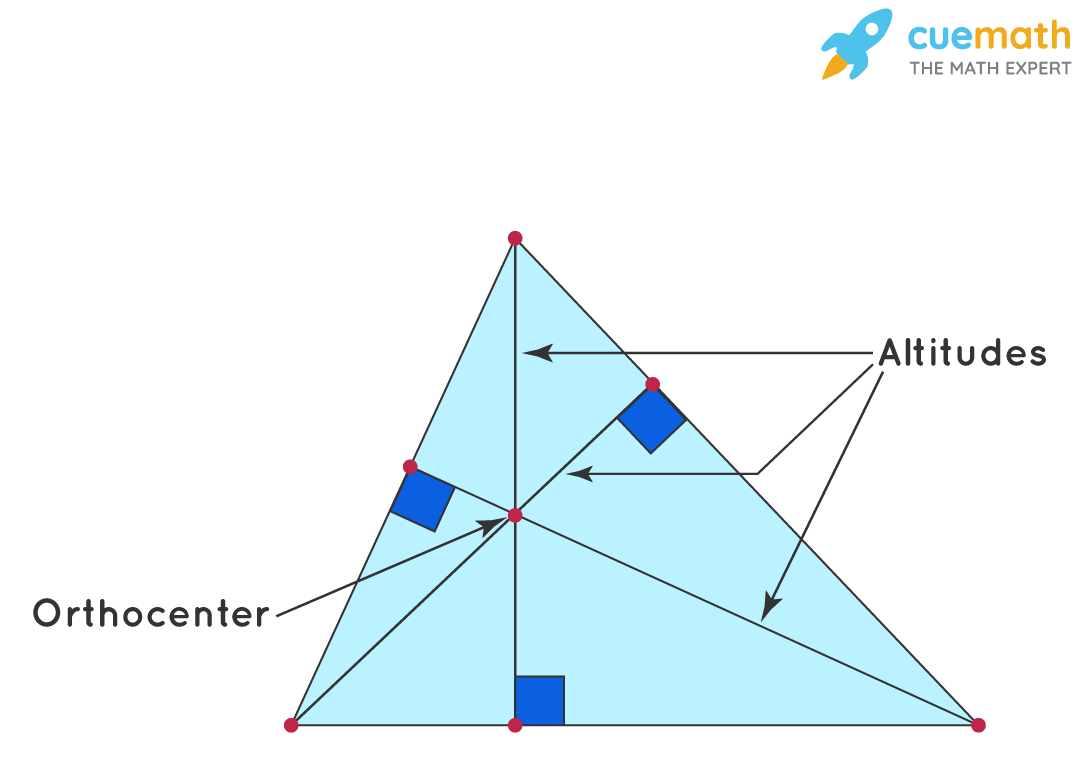# What Is the Altitude of a Triangle

## Question: What is the altitude of a triangle?

The triangle is a polygon with three vertices and three edges.

## Answer: A perpendicular that is drawn from the vertex of a triangle to the opposite side is called the altitude of a triangle.

Let us explore the topic altitude in detail.

## Explanation:

The altitude of a triangle is the perpendicular line segment drawn from the vertex of the triangle to the side opposite to it. It is also known as the height or the perpendicular of the triangle.

There are three altitudes in every triangle drawn from each of the vertices. The point where all the three altitudes meet inside a triangle is known as the Orthocenter.The altitude of a triangle is the perpendicular distance from the base to the opposite vertex. It can be both outside or inside the triangle depending on the type of the triangle.